### 1 APPENDIX: A Tutorial On Logarithms

Understanding the Log Function. In the mathematical operation of addition we take two numbers and join them to create a third: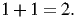(21)

We can repeat this operation: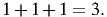(22)

Multiplication is the mathematical operation that extends this: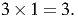(23)

In the same way, we can repeat multiplication: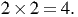(24)

and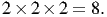(25)

The extension of multiplication is exponentiation: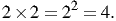(26)

and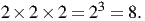(27)

This is read “two raised to the third is eight”. Because exponentiation simply counts the number of multiplications, the exponents add: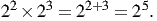(28)

The number ‘2’ is called the base of the exponentiation. If we raise an exponent to another exponent, the values multiply: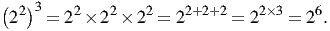(29)

The exponential function y = 2x is shown in this graph7 :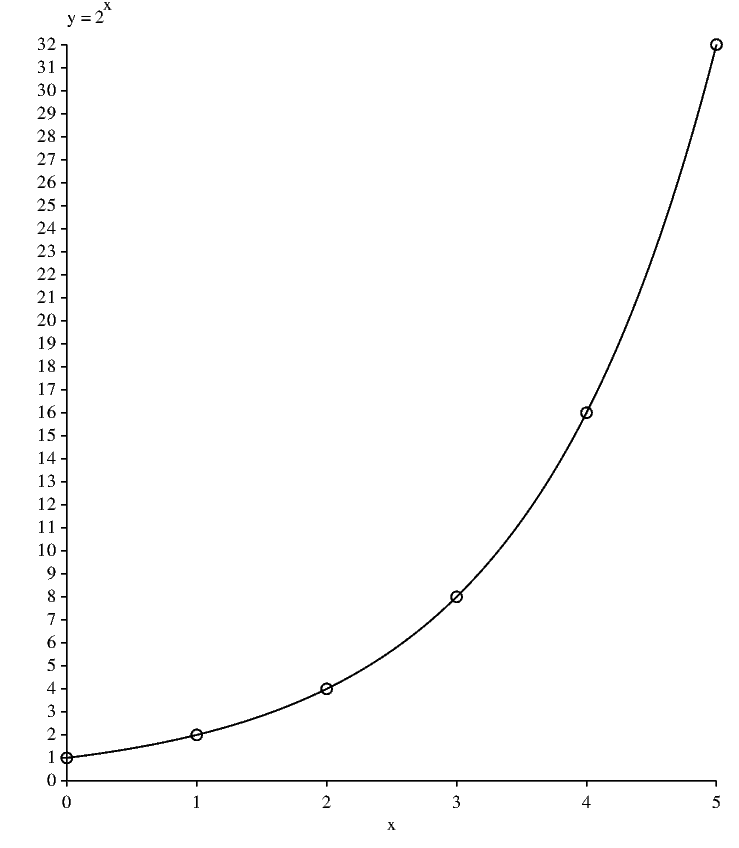Figure 1: The exponential function.

Now consider that we have a number and we want to know how many 2’s must be multiplied together to get that number. For example, given that we are using ‘2’ as the base, how many 2’s must be multiplied together to get 32? That is, we want to solve this equation: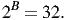(30)

Of course, 25 = 32, so B = 5. To be able to get a hold of this, mathematicians made up a new function called the logarithm: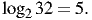(31)

We pronounce this as “the logarithm to the base 2 of 32 is 5”. It is the “inverse function” for exponentiation: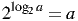(32)

and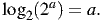(33)

The logarithmic function y = log2x is shown in this graph8 :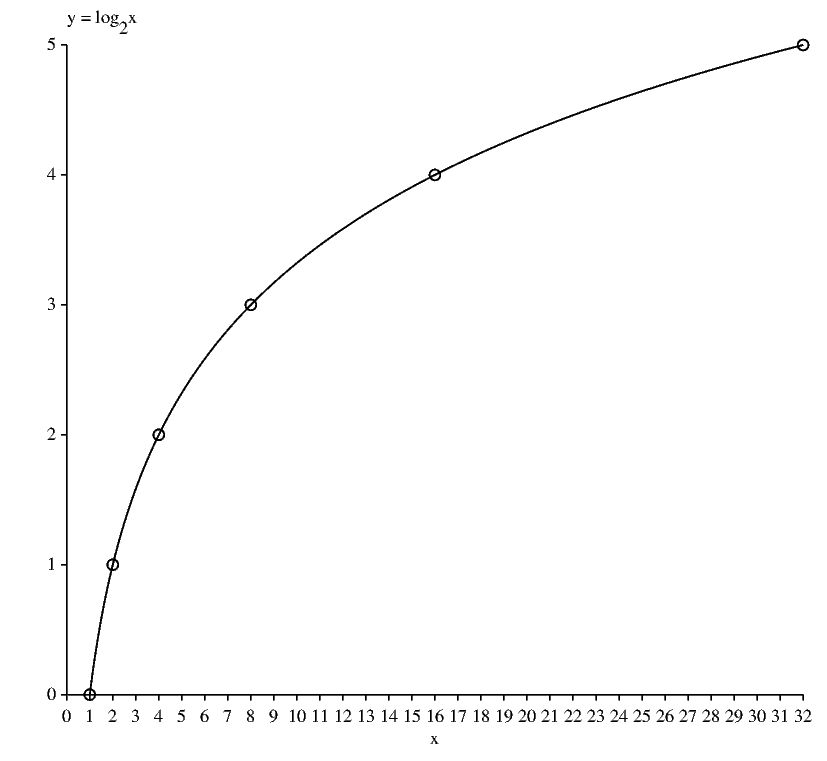Figure 2: The logarithmic function.

This graph was created by switching the x and y of the exponential graph, which is the same as flipping the curve over on a 45 line. Notice in particular that log2(1) = 0 and log2(0) = -∞.

The Addition Law. Consider this equation: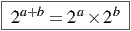(34)

which is just a generalization of equation (28). Take the logarithm of both sides: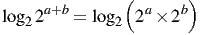(35)

Exponentiation and the logarithm are inverse operations, so we can collapse the left side: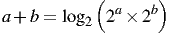(36)

Now let’s be tricky and substitute: log2x = a and log2y = b: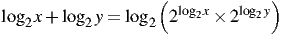(37)

Again, exponentiation and the logarithm are inverse operations, so we can collapse the two cases on the right side: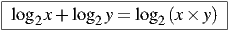(38)

This is the additive property that Shannon was interested in.

The ‘Pull Forward’ Rule. From equation (32):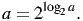(39)

Raise both sides to the u: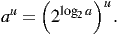(40)

Now, we can combine the exponents by multiplying, as in (29):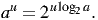(41)

Finally, take the log base 2 of both sides and collapse the right side: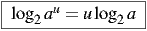(42)

This can be remembered as a rule that allows one to “pull” the exponent forward from inside the logarithm.

How to Convert Between Different Bases. Calculators and computers generally don’t calculate the logarithm to the base 2, but we can use a trick to make this easy. Start by letting: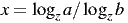(43)

Rearrange it as: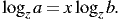(44)

Now use a “reverse pull forward” (!):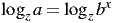(45)

and drop the logs: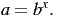(46)

Now take the log base b: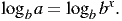(47)

This simplifies to: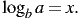(48)

But we know what x is from equation (43):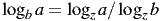(49)

The conversion rule to get logarithms base b = 2 from any base z is: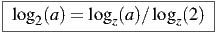(50)

Notice that since the z does not appear on the left hand side it doesn’t matter what kind of logarithm you have available, because you can always get to another base using this equation! Try this example on your calculator: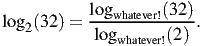(51)

You should get ‘5’.

Tricks With Powers of 2. In calculus we learn about the natural logarithm with base e = 2.7182818284590459 Calculations with this base can easily be done by a computer or calculator, but they are difficult for most people to do in their head.

In contrast, the powers of 2 are easy to memorize and remember:

 choices bits M B 1 0 2 1 4 2 8 3 16 4 32 5 64 6 128 7 256 8 512 9 1024 10

where 2B = M and log2M = B.

We can use this table and a trick to make quick estimates of the logs of higher numbers. Notice that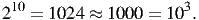(52)

So to take the log base 2 of 4×106, we think: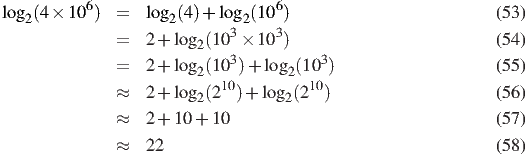The actual value is 21.93.